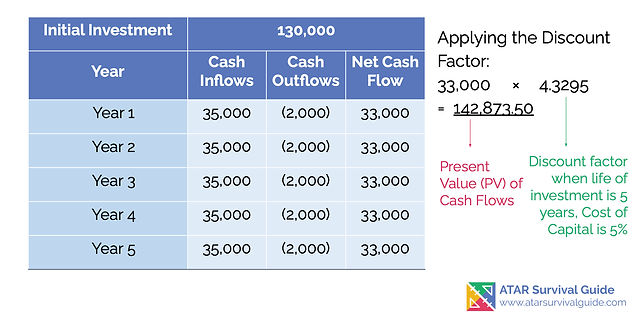### Capital Expenditure

#### NPV with Annuity Cash Flows

###### Content ContributorsPriya KaurChristian Bien

# Learning Objectives###### ​###### What is the Net Present Value and How is it Calculated?The net present value is the amount resulting from discounting the net cash flows (cash inflows - cash outflows) at the minimum discount rate to their present values, and then subtracting the present value cost of the Capital Investment.

Net Present Value = (PV of Future Cash Flows) - (PV Cost of Capital Investment) According to the Decision Rule, a positive NPV result indicates the Capital Investment is acceptable, and a negative NPV result means the Capital Investment should be rejected.###### Worked Example: Simple Net Present ValueBelow is an example of how to calculate the Net Present Value for a capital investment that will generate the same amount of net cash flows each year. This is known as an Annuity. Bob Builders Pty Ltd, requires purchase of a new truck which will cost \$130,000.

The Company estimates that the truck will produce economic benefits of \$35,000 per year, and economic outflows of \$2000 per year. The Truck is estimated to have a useful life of 5 years, and the Cost of Capital is 5%. Calculate the NPV for the Investment of the New Truck and determine if Bob Builders should go ahead with this Investment.###### Step 1: Calculate the Net Cash FlowsThe NPV table has been extended to include an additional column that calculates the Net Cash Flows for each year - which results from that year's inflows subtracting the outflows for that same year.

E.g. At Year 1: \$35,000 (Inflows) - \$2000 (Outflows)= \$33,000 (Net Cash Flow). We can see that the Net Cash Flows are the same each year, therefore we can use the Annuity method of NPV calculation.###### Step 2: Applying the Annuity Discount MethodAs stated above, since the Net Cash Flows are the same each, the annuity method of NPV Calculation can be applied. To do this, the Net Cash Flow for ONE Period is multiplied with the discount factor. From the question we know the truck has a useful life of 5 years and the Cost of Capital is 5%.

Therefore, the discount factor to be used is '4.3295' (refer to the image at the bottom of this page). = 33,000 × 4.3295 = 142,873.50 ----> This figure is known as the Present Value of Future Cash Flows. * For all Annuities, the discount factors should be attained from the 'Present value of an ordinary annuity of \$1 at the end of future periods' table provided in the Accounting and Finance ATAR Specifications

Booklet which can be found here: https://senior-secondary.scsa.wa.edu.au/__data/assets/pdf_file/0012/593895/2020_ACF_Specification_Booklet.PDF.###### Step 3: Finding the Net Present Value of the Capital InvestmentAs stated above, the formula for the Net Present Value = (PV of Future Cash Flows) - (PV Cost of Capital Investment).

In Step 2, we have calculated the Present Value of Future Cash Flows to be = 142,873.50. From the question we are told the cost of the truck is \$130,000. By substituting these figures in the NPV Formula: = 142,873.50 (PV of Future Cash Flows) - 130,000 (PV Cost of Capital Investment) =\$ 12,873.50. This is the Net Present Value.###### Step 4: Interpretation of the Net Present ValueThe final NPV is a positive figure of \$ 12,873.50.

This can be interpreted as the truck investment will make \$ 12,873.50 more than the minimum 5% return expected from the Cost of Capital, therefore this investment should be Accepted.###### Reference: Discount Factor Table in the Case of AnnuitiesThe image of the table is extracted from the Specifications booklet.

From the question we know the truck has a useful life of 5 years and the Cost of Capital is 5%. Therefore, the discount factor to be used is '4.3295'.###### ​###### NPV with Differing Cash Flows#### Registrations Now Open for Empowered AcademyA Free Student-Centred Revision Program